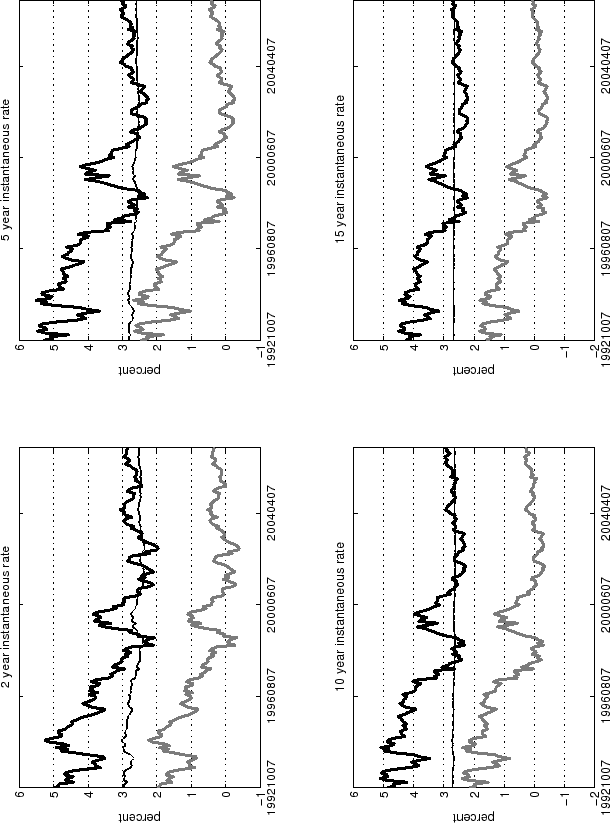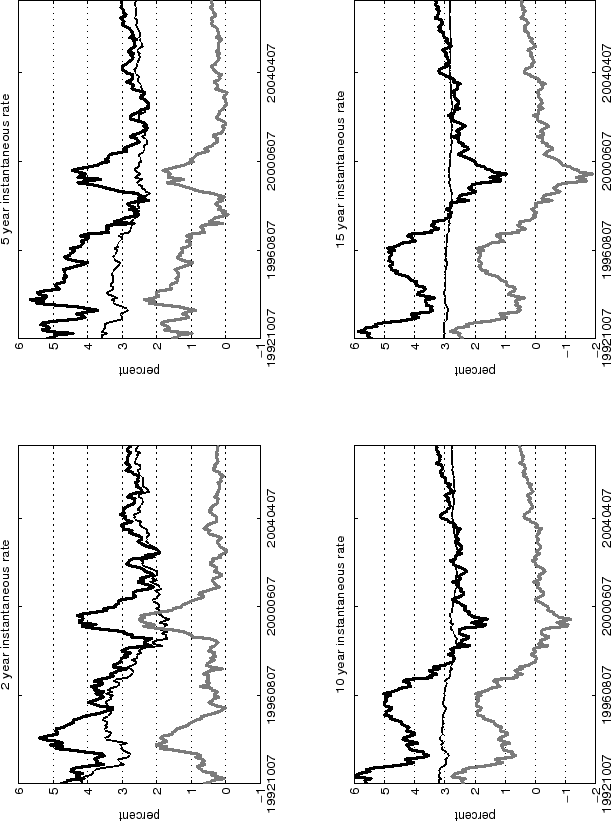# Gaussian factor models futures and forward prices

Post on: 29 Апрель, 2016Gaussian Factor Models — Futures and Forward Prices

Cody. B. Hyndman

Department of Mathematics and Statistics, Concordia University

1455 boulevard de Maisonneuve Ouest

Montr´ eal, Qu´ ebec

email: hyndman@mathstat.concordia.ca

March 10, 2007

Abstract

We completely characterise the futures price and forward price of a risky asset (commodity) paying

a stochastic dividend yield (convenience yield). The asset (commodity) price is modelled as an expo-

nential affine function of a Gaussian factors process while the interest rate and dividend yield are affine

functions of the factors process. The characterisation we provide is based on the method of stochastic

flows. We believe this method leads to simpler and more clear-cut derivations of the futures price and

forward price formulae than alternative methods. Hedging a long term forward contract with shorter

term futures contracts and bonds is also examined.

Keywords: futures price; forward price; stochastic flows; factor models; Gaussian state variables

The author would like to thank Andrew Heunis and Robert Elliott for helpful discussions.

The author would like to acknowledge the financial support of MITACS through the “Prediction in Interacting Sys-

tems (PINTS)” and “Modelling Trading and Risk in the Market” projects and the financial support of the Institut de finance

math´ ematique de Montr´ eal (IFM2).

Page 2

Gaussian Factor Models — Futures and Forward Prices

1 Introduction

Gaussian factormodels of asset prices have been extensively used in financial modelling. Gaussian models

remain popular due to their analytic tractability and well established statistical methodology. Continuous-

time models for futures and forward prices have been studied by Gibson and Schwartz (1990), Schwartz

(1997, 1998), Cortazar and Schwartz (1994), Miltersen and Schwartz (1998), Schwartz and Smith (2000),

and Manoliu and Tompaidis (2002) among others. The work of Miltersen and Schwartz (1998) is notable

in that it develops an analogue of the Heath, Jarrow and Morton (1992) model in the context of futures

markets. Other general studies on futures and forward prices include Schroder (1999), Bj¨ ork and Land´ en

(2002), and the references contained therein.

In this paper we completely characterise the futures price and forward price of a risky asset (commodity)

paying a stochastic dividend yield (convenience yield). The interest rate and dividend yield are modelled

as affine functions of a Gaussian factors process. We also assume that the asset price is modelled as

an exponential affine function of the Gaussian factors process. Our analysis is based on the method

of stochastic flows introduced to the term structure literature by Elliott and van der Hoek (2001). The

stochastic flows method presented in this paper includes the Gaussian factors models which have appeared

in the literature as a special case and has been generalized to include models which include non-Gaussian

(square-root or Cox, Ingersoll and Ross (1985) type) factors. One of the main contributions of this paper

is a unified framework under which to study a wide class of models in a systematic and clear-cut way.

Most of the derivations of futures and forward prices which have appeared in the literature, with the excep-

tion of Bj¨ ork and Land´ en (2002), have been model specific and do not address the entire class of Gaussian

factor models. Derivations of futures and forward prices that have appeared in the literature have usually

been based on solving a partial differential equation (PDE) or calculating a conditional expectation using

the distributional properties of the factors process. However, in the case of PDE derivations the exponen-

tial affine form of the solution is guessed and then substituted into the PDE to reduce the problem to the

solution of ordinary differential equations (ODEs). Our method shows why the solution is exponential

affine by characterizing the futures and forward price as the solution to a linear ODE. Derivations of the

prices based on distributional properties of the factors process, for example Bjerksund (1991), are often

complicated when some factors are correlated. Further, our method has been generalised to study other

models, outside the assumptions of this paper, where the functional form of the solution to the PDE forthe contingent claim is not easily guessed.

Page 3

Gaussian Factor Models — Futures and Forward Prices

Due to certain shortcomings of Gaussian models, or the desire to better model asset price movements,

more complicated models which often incorporate non-Gaussian components and jumps have become

widely used. For example Yan (2002), Bj¨ ork and Land´ en (2002), and Ribeiro and Hodges (2004) em-

ploy combinations of Gaussian, non-Gaussian, and jump factors. For non-Gaussian factor models certain

approximations and assumptions, often left unjustified, are made to allow for a practical implementation

of the model or to calibrate the model to historical data. For models containing a combination of Gaus-

sian and Cox, Ingersoll and Ross (1985) type factors the validity of applying the Feynman-Kac formula

to derive derivative prices has only been recently justified by Levendorski˘ ı (2004) despite the previous

appearance of such models and derivations in the literature.

The methods of this paper have been extended to ‘affine term structure models’ and ‘affine price mod-

els’ in Hyndman (2005) where the factors process follows an affine diffusion. This extension includes

as special cases the continuous factors model of Duffie and Kan (1996) for bond prices and Bj¨ ork and

Land´ en (2002) for futures and forward prices. Additional tools, namely changes of measure and forward-

backward stochastic differential equations, are required to derive the bond, futures, and forward prices for

general affine factor models which are not required in the Gaussian case. Therefore, we shall concentrate

exclusively on the Gaussian case in this paper.

The remainder of this paper is organised as follows. Section 2 discusses the market model and sets some

notation. Section 3 studies the futures price, Section 4 reviews some results on the bond price which are

necessary for our discussion of the forward price in Section 5, and Section 6 examines hedging a long

term forward commitment using shorter term futures contracts and bonds. Section 7 briefly discusses

implementation and the estimation of model parameters, Section 8 shows how our methods apply to three

different commodity market models that have appeared in the literature, and Section 9 concludes.

2 Market Model

Let (Ω,F ,P) be a complete probability space on which there is given a standard, m-dimensional Brownian

More from this section
Categories
Tags

• Most Popular